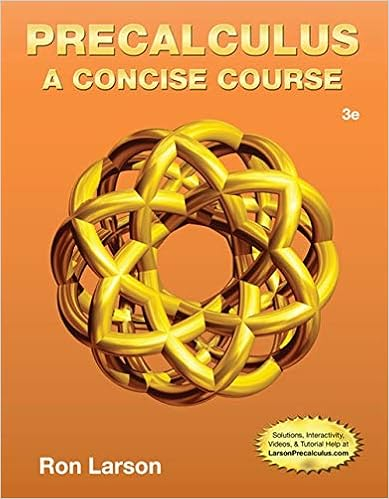# 2.9 Practice Prelim Questions - Review Problems for Prelim...

• Notes
• 2

This preview shows page 1 - 2 out of 2 pages.

##### We have textbook solutions for you!
The document you are viewing contains questions related to this textbook.The document you are viewing contains questions related to this textbook.
Chapter 2 / Exercise 83
Precalculus: A Concise Course
LarsonExpert Verified
Review Problems for Prelim 2 Maxima, minima, convexity, concavity (a) Find all the critical points. (b) Use the second derivative test to determine if they are local maxima or local minima. Find the open intervals on which the function is (c) increasing, (d) convex. 1. x 3 + 3 x 2 - 9 x + 6 2. x 3 / 3 - 5 x 2 / 2 + 4 x 3. x - 6 x + 2 ln x , for x > 0 4. x 8 / 5 + x - 2 / 5 for x > 0. 5. x ln x for x > 0 6. xe x 7. xe - x 2 / 2 8. Consider the function f ( x ) = x/ (4+ x 2 ). Use the first derivative test to find the maximum value for x 0. 9. At what point is the functionf(x) =-x3+x2+x+ 1 increasing the fastest? 10. The San Diego zoo wants to build a new enclosure for birds. The base will be an r × r square and the height will be h . The bottom will be on the ground but they need to use material for the top and the four sides. Suppose they want to enclose 4000 cubic feet of space and that the cost is proportion to the area of fencing needed to enclose the cage. How
##### We have textbook solutions for you!
The document you are viewing contains questions related to this textbook.The document you are viewing contains questions related to this textbook.
Chapter 2 / Exercise 83
Precalculus: A Concise Course
LarsonExpert Verified
•••